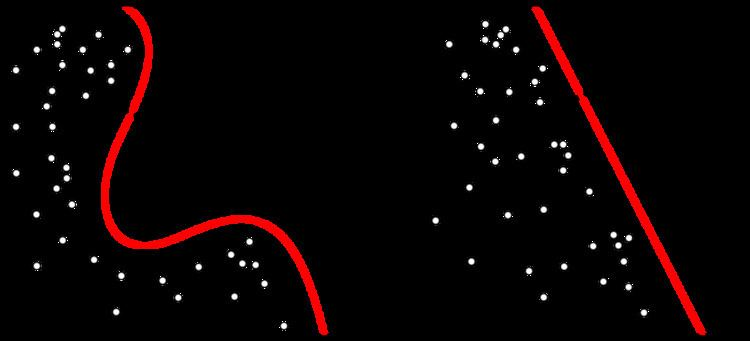# Temporal difference learning

Updated onTemporal difference (TD) learning is a prediction-based machine learning method. It has primarily been used for the reinforcement learning problem, and is said to be "a combination of Monte Carlo ideas and dynamic programming (DP) ideas." TD resembles a Monte Carlo method because it learns by sampling the environment according to some policy, and is related to dynamic programming techniques as it approximates its current estimate based on previously learned estimates (a process known as bootstrapping). The TD learning algorithm is related to the temporal difference model of animal learning.

## Contents

As a prediction method, TD learning considers that subsequent predictions are often correlated in some sense. In standard supervised predictive learning, one learns only from actually observed values: A prediction is made, and when the observation is available, the prediction mechanism is adjusted to better match the observation. As elucidated by Richard Sutton, the core idea of TD learning is that one adjusts predictions to match other, more accurate, predictions about the future. This procedure is a form of bootstrapping, as illustrated with the following example:

"Suppose you wish to predict the weather for Saturday, and you have some model that predicts Saturday's weather, given the weather of each day in the week. In the standard case, you would wait until Saturday and then adjust all your models. However, when it is, for example, Friday, you should have a pretty good idea of what the weather would be on Saturday - and thus be able to change, say, Monday's model before Saturday arrives."

Mathematically speaking, both in a standard and a TD approach, one would try to optimize some cost function, related to the error in our predictions of the expectation of some random variable, E[z]. However, while in the standard approach one in some sense assumes E[z] = z (the actual observed value), in the TD approach we use a model. For the particular case of reinforcement learning, which is the major application of TD methods, z is the total return and E[z] is given by the Bellman equation of the return.

## Mathematical formulation

Let r t be the reward (return) on time step t. Let V ¯ t be the correct prediction that is equal to the discounted sum of all future reward. The discounting is done by powers of factor of γ such that reward at distant time step is less important.

V ¯ t = i = 0 γ i r t + i

where 0 γ < 1 . This formula can be expanded

V ¯ t = r t + i = 1 γ i r t + i

by changing the index of i to start from 0.

V ¯ t = r t + i = 0 γ i + 1 r t + i + 1 V ¯ t = r t + γ i = 0 γ i r t + i + 1 V ¯ t = r t + γ V ¯ t + 1

Thus, the reward is the difference between the correct prediction and the current prediction.

r t = V ¯ t γ V ¯ t + 1

## TD-Lambda

TD-Lambda is a learning algorithm invented by Richard S. Sutton based on earlier work on temporal difference learning by Arthur Samuel. This algorithm was famously applied by Gerald Tesauro to create TD-Gammon, a program that learned to play the game of backgammon at the level of expert human players.

The lambda ( λ ) parameter refers to the trace decay parameter, with 0 λ 1 . Higher settings lead to longer lasting traces; that is, a larger proportion of credit from a reward can be given to more distant states and actions when λ is higher, with λ = 1 producing parallel learning to Monte Carlo RL algorithms.

## TD algorithm in neuroscience

The TD algorithm has also received attention in the field of neuroscience. Researchers discovered that the firing rate of dopamine neurons in the ventral tegmental area (VTA) and substantia nigra (SNc) appear to mimic the error function in the algorithm. The error function reports back the difference between the estimated reward at any given state or time step and the actual reward received. The larger the error function, the larger the difference between the expected and actual reward. When this is paired with a stimulus that accurately reflects a future reward, the error can be used to associate the stimulus with the future reward.

Dopamine cells appear to behave in a similar manner. In one experiment measurements of dopamine cells were made while training a monkey to associate a stimulus with the reward of juice. Initially the dopamine cells increased firing rates when the monkey received juice, indicating a difference in expected and actual rewards. Over time this increase in firing back propagated to the earliest reliable stimulus for the reward. Once the monkey was fully trained, there was no increase in firing rate upon presentation of the predicted reward. Continually, the firing rate for the dopamine cells decreased below normal activation when the expected reward was not produced. This mimics closely how the error function in TD is used for reinforcement learning.

The relationship between the model and potential neurological function has produced research attempting to use TD to explain many aspects of behavioral research. It has also been used to study conditions such as schizophrenia or the consequences of pharmacological manipulations of dopamine on learning.

Topics